﻿ A Double Inequality for the Harmonic Number in Terms of the Hyperbolic Cosine

### A Double Inequality for the Harmonic Number in Terms of the Hyperbolic Cosine

Da-Wei Niu, Yue-Jin Zhang, Feng QiOPEN ACCESSPEER-REVIEWED

## A Double Inequality for the Harmonic Number in Terms of the Hyperbolic Cosine

Da-Wei Niu1,, Yue-Jin Zhang1, Feng Qi2, 3, 4

1College of Information and Business, Zhongyuan University of Technology, Zhengzhou City, Henan Province, China

2College of Mathematics, Inner Mongolia University for Nationalities, Tongliao City, Inner Mongolia Autonomous Region, China

3Department of Mathematics, College of Science, Tianjin Polytechnic University, Tianjin City, China

4Institute of Mathematics, Henan Polytechnic University, Jiaozuo City, Henan Province, China

### Abstract

In the paper, the author present an inequality for bounding the harmonic number in terms of the hyperbolic cosine.

• Niu, Da-Wei, Yue-Jin Zhang, and Feng Qi. "A Double Inequality for the Harmonic Number in Terms of the Hyperbolic Cosine." Turkish Journal of Analysis and Number Theory 2.6 (2014): 223-225.
• Niu, D. , Zhang, Y. , & Qi, F. (2014). A Double Inequality for the Harmonic Number in Terms of the Hyperbolic Cosine. Turkish Journal of Analysis and Number Theory, 2(6), 223-225.
• Niu, Da-Wei, Yue-Jin Zhang, and Feng Qi. "A Double Inequality for the Harmonic Number in Terms of the Hyperbolic Cosine." Turkish Journal of Analysis and Number Theory 2, no. 6 (2014): 223-225.

 Import into BibTeX Import into EndNote Import into RefMan Import into RefWorks

### 1. Introduction

The harmonic numberis defined as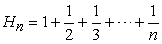and it has the following close connections with the Euler-Mascheroni constant: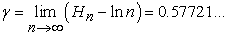andwhereis the digamma function which is the logarithmic derivative of the classical Euler gamma functionThe harmonic number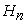has interesting applications in many areas of mathematics, such as number theory, special functions, and combinatorics. For example, Lagarias proved that the Riemann hypothesis is equivalent to the statement thatfor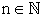, wheredenotes the sum of the divisors of n.

In , Paule and Schneider obtained the identity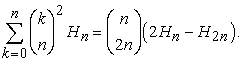In , Alzer presented the inequality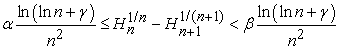for, where the constants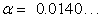andare the best possible. In , Batir gave an inequalityThis double inequality was refined in  by replacing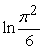by 1. It also inspired Mortici to construct a sequence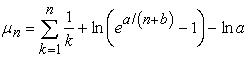in , which converges to more quickly.

For more information on the harmonic number, please refer to [2,6-19,21-26] and plenty of references therein.

In this paper, we will establish a new double inequality for bounding the harmonic numberin terms of the hyperbolic cosine.

Our main result may be stated as the following theorem.

Theorem 1.1. For all positive integers, we have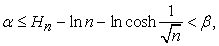(1.1)

where the constantsandare the best impossible.

### 2. Lemmas

In order to prove Theorem 1.1, we need the following lemmas.

Lemma 2.1 ([, p. 384]). Letand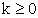be integers, for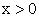, we have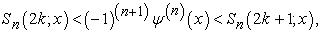whereLemma 2.2 ([10, 22]). For, we have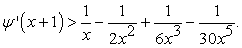(2.1)

### 3. Proof of Theorem 1.1

Now we are in a position to prove our Theorem 1.1.

LetA direct differentiation yields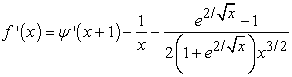and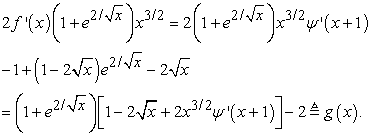By virtue of inequalities (2.1) and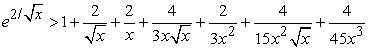we acquirefor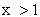. This implies thatand that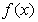is increasing on.

By the asymptotic expansion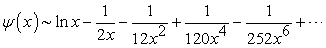asin [, p. 259] and the well-known formula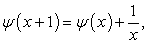(3.1)

we easily find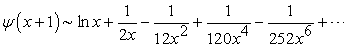as. Hence, it follows thatTaking into account thatis increasing onreveals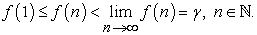(3.2)

Combining (1), (3.1), and (3.2) concludes that the double inequality (1.1) holds for all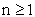and that the bounds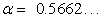andin (1.1) are the best impossible. The proof of Theorem 1.1 is complete.

### References

  M. Abramowitz and I. A. Stegun (Eds), Handbook of Mathematical Functions with Formulas, Graphs, and Mathematical Tables, National Bureau of Standards, Applied Mathematics Series 55, 10th printing, Washington, 1972.In article  H. Alzer, Inequalities for the harmonic numbers, Math. Z. 267 (2011), no. 1-2, 367-384.In article CrossRef  H. Alzer, On some inequalities for the gamma and psi functions, Math. Comp. 66 (1997), no. 217, 373-389.In article CrossRef  H. Alzer, Sharp inequalities for the harmonic numbers, Expo. Math. 24 (2006), no. 4, 385-388.In article CrossRef  N. Batir, Some new inequalities for gamma and polygamma functions, J. Inequal. Pure Appl. Math. 6 (2005), no. 4, Art. 103.In article  C.-P. Chen, Inequalities for the Euler-Mascheroni costant, Appl. Math. Lett. 23 (2010), no. 2, 161-164.In article CrossRef  C.-P. Chen, Sharpness of Negoi's inequality for the Euler-Mascheroni constant, Bull. Math. Anal. Appl. 3 (2011), no. 1, 134-141.In article  C.-P. Chen and C. Mortici, New sequence converging towards the Euler-Mascheroni constant, Comp. Math. Appl. 64 (2012), no. 2, 391-398.In article CrossRef  D. W. DeTemple, A quicker convergence to Euler's constant, Amer. Math. Monthly 100 (1993), no. 5, 468-470.In article  B.-N. Guo and F. Qi, Two new proofs of the complete monotonicity of a function involving the psi function, Bull. Korean Math. Soc. 47 (2010), no. 1, 103-111.In article CrossRef  B.-N. Guo and F. Qi, Sharp bounds for harmonic numbers, Appl. Math. Comput. 218 (2011), no. 3, 991-995.In article CrossRef  B.-N. Guo and F. Qi, Sharp inequalities for the psi function and harmonic numbers, Analysis (Berlin) 34 (2014), no. 2, 201-208.In article CrossRef  E. A. Karatsuba, On the computation of the Euler constant, Numer. Algor. 24 (2000), no. 1-2, 83-97.In article CrossRef  W.-H. Li, F. Qi, and B.-N. Guo, On proofs for monotonicity of a function involving the psi and exponential functions, Analysis (Munich) 33 (2013), no. 1, 45-50.In article CrossRef  C. Mortici, A quicker convergence toward the constant with the logarithm term involving the constant e, Carpathian J. Math. 26 (2010), no. 1, 86-91.In article  C. Mortici, Improved convergence towards generalized Euler-Mascheroni constant, Appl. Math. Comput. 215 (2010), no. 9, 3443-3448.In article CrossRef  C. Mortici, New approximations of the gamma function in terms of the digamma function, Appl. Math. Lett. 59 (2010), no. 1, 97-100.In article CrossRef  C. Mortici, On new sequences converging towards the Euler-Mascheroni constant, Comput. Math. Appl. 59 (2010), no. 8, 2610-2614.In article CrossRef  T. Negoi, A faster convergence to Euler's constant, Math. Gaz. 83 (1999), no. 498, 487-489.In article  P. Paule and C. Schneider, Computer proofs of a new family of harmonic number identities, Adv. Appl. Math. 31 (2003), no. 2, 359-378.In article CrossRef  F. Qi, Complete monotonicity of functions involving the q-trigamma and q-tetragamma functions, Rev. R. Acad. Cienc. Exactas Fís. Nat. Ser. A Math. RACSAM. 109 (2015), in press.In article CrossRef  F. Qi, R.-Q. Cui, C.-P. Chen and B.-N. Guo, Some completely monotonic functions involving polygamma functions and an application, J. Math. Anal. Appl. 310 (2005), no. 1, 303-308.In article CrossRef  F. Qi and Q.-M. Luo, Complete monotonicity of a function involving the gamma function and applications, Period. Math. Hungar. 69 (2014), no. 2, 159-169.In article CrossRef  A. Sîntǎmǎrian A generalization of Euler's constant, Numer. Algor. 46 (2007) no. 2, 141-151.In article CrossRef  M. B. Villarino, Ramanujan's harmonic number expansion into negative powers of a triangular num- ber, J. Inequal. Pure Appl. Math. 9 (2008), no. 3, Art. 89.In article  R. M. Young, Euler's constant, Math. Gaz. 75 (1991), 187-190.In article CrossRef